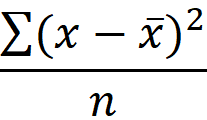The VARPA function is a statistical function that can calculate and return the variance of a population by evaluating text and logical values. Variance provides a general idea of how much data points are spread in a data set, and can be used to calculate the standard deviation or determine the risk of an investment. In this guide, we’re going to show you how to use the VARPA function and also go over some tips and error handling methods.

## Supported versions

• Excel 2003 and newer versions

## VARPA Function Syntax

VARPA(number1,[number2],...)

## Arguments

 number1 The first number argument corresponding to the entire population. [number2] Optional. Other arguments corresponding to the entire population. Up to 254 can be added.

## VARPA Function Example

The function accepts numeric values as its arguments. Any type of text or logical values will be ignored. You can use range references or static values just like in any other formula.

The function calculates the variance using the following function:• x: population mean (average)
• x ̅: element of the population
• n: population size

Here is the comparison of the two approaches:

=VARPA(population)

=LET(evaluated,IF(ISNUMBER(--sample),--sample,0),

SUMPRODUCT(POWER(AVERAGE(evaluated)-evaluated,2))/

COUNT(evaluated))

The formulas are using the named range population (B5:B10).The example uses the LET function to simplify the mathematical approach. Check out the Function: LET article to learn more.

## Tips

• The VARPA function assumes that its arguments represent the entire population. If your data is a sample of the population, use VARA.
• The VARPA function evaluates text and logical values like TRUE and FALSE. If you want to ignore non-numeric values, use the VAR.P function instead.
• Empty cells are ignored.

## Issues

• Any error in the arguments will cause the function to return an error.